# PSEB 7th Class Maths Solutions Chapter 10 Practical Geometry Ex 10.1

Punjab State Board PSEB 7th Class Maths Book Solutions Chapter 10 Practical Geometry Ex 10.1 Textbook Exercise Questions and Answers.

## PSEB Solutions for Class 7 Maths Chapter 10 Practical Geometry Ex 10.1

1. Draw a line, l, take a point p outside it. Through p, draw a line parallel to l using ruler and compass only.
Solution:
Steps of Construction :
Step 1. Draw a line l of any suitable length Mid a point ‘p’ outside l [see Fig. (i)].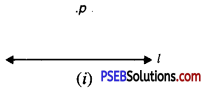Step 2. Take a point ‘q’ on l and join q to p [see Fig. (ii)].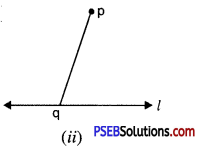Step 3. With q as centre and a convenient radius, draw an arc cutting l at E and pq at F [see Fig. (iii)].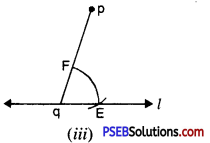Step 4. Now with p as a centre and the same radius as in step 3, draw an arc GH cutting pq at I [see Fig. (iv)].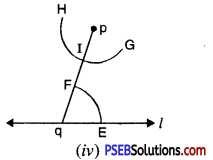Step 5. Place the pointed tip of the compasses at E and adjust the opening so that the pencil tip is at F [see Fig. (v)].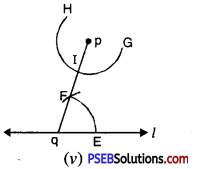Step 6. With the same opening as in step 5 and with I as centre, draw an arc cutting the arc GH at J. [see Fig. (vi)].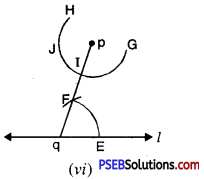Step 7. Now, join pand J to draw a line ‘m’ [see Fig. (vii)],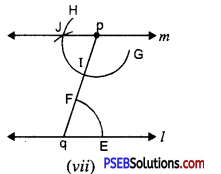Note that : ∠Jpq and ∠pqE are alternate interior angles and ∠pqE = ∠qpJ
∴ m || l

2. Draw a line parallel to a line l at a distance of 3.5 cm from it.
Solution :
Steps of construction :
Step 1. Take a line ‘l’ and any point say O on it [see Fig. (i)].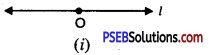Step 2. At O draw ∠AOB = 90°. [see Fig. (ii)].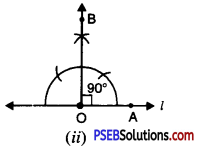Step 3. Place the pointed tip of the compasses at ‘0’ (zero) mark on ruler and adjust the opening so that the pencil tip is at 3.5 cm [see Fig. (iii)]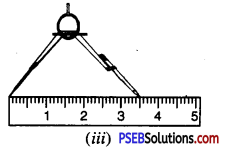Step 4. With the same opening as in step 3 and with O as centre draw an arc cutting ray OB at X. [see Fig. (iv)].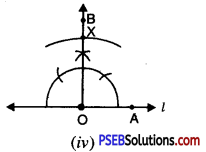Step 5. At X draw a line ‘m’ perpendicular to OB. In other words, draw ∠CXO = 90° [see Fig. (v)].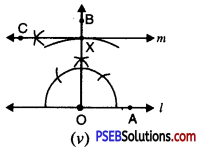In this way, line m is parallel to l.
Note that. ∠AOX and ∠CXO are alternate angles and ∠AOX = ∠CXO (each = 90°).
∴ m || l.
Note. We may use any of three properties regarding the transversal OX and parallel lines l and m.

3. Let l be a line and P be a point not on l. Through P, draw a line ‘m’ parallel to l. Now, join P to any point Q on l. Choose any other point R on m. Through R, draw a line parallel to PQ. Let this meets l at S. What shape do the two sets of parallel lines enclose ?
Solution:
Steps of Construction :
Step 1. Take a line ‘l’ and a point ‘P’ outside l. [see Fig . (i)]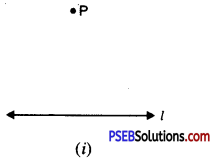Step 2. Take any point A on l and join P to A [see Fig. (ii)]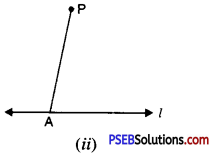Step 3. With A as centre and convenient radius draw an arc cutting l at B and AP at C. [see Fig. (iii)]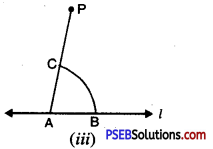Step 4. Now with P as centre and the same radius as in step 3, draw an arc DE cutting PA at F [see Fig. (iv)].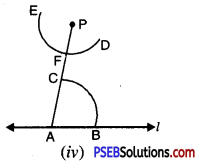Step 5. Place the pointed tip of the compasses at B and adjust the opening so that the pencil tip is at C [see Fig. (v)].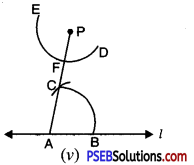Step 6. With the same opening as in step 5 and with F as centre, draw an arc cutting the arc DE at G [see Fig. (vi)].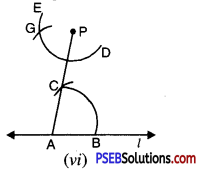Step 7. Now join PG to draw line ‘m’ [see Fig. (vii)].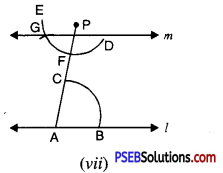Note that. ∠PAB and ∠APG are alternate interior angles and ∠PAB = ∠APG
∴ m || l
Step 8. Take any point Q on l. Join PQ [see Fig. (viii)].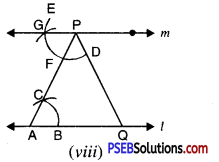Step 9. Take any other point R on m [see Fig. (ix)].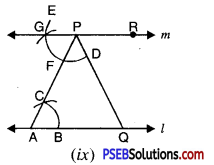Step 10. With P as centre and convenient radius, draw an arc cutting line m at H and PQ at I [see Fig. (x)].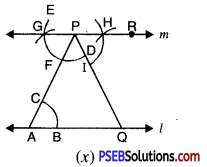Step 11. Now with R as centre and the same radius as in step 10, draw an arc JK [see Fig. (xi)].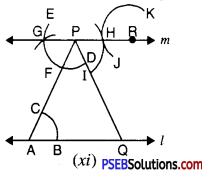Step 12. Place the pointed tip of compasses at H and adjust the opening so that the pencil tip is at I.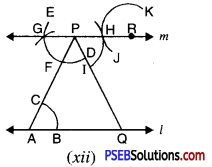Step 13. With the same opening as in step 12 and with R as centre, draw an arc cutting the arc JK at L [see Fig. (xii)].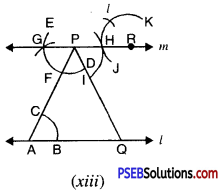Step 14. Now join RL to draw a line parallel to PQ. Let this meet l at S. [see Fig. (xiv)]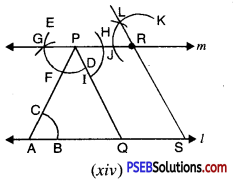Note that. ∠RPQ and ∠LRP are alternate interior angles
and ∠RPQ = ∠LRP
∴ RS || PQ.
Now we have
PR || QS
[∵ m || l and PR is part of m and QS is part of line l]
and PQ || RS
∴ PQSR is a parallelogram.4.

Question (i).
How many parallel lines can be drawn, passing through a point not lying on the given line ?
(a) 0
(b) 2
(c) 1
(d) 3